Dr. Ahed Habib

# Practical Analysis of Wind Load for Residential Structures to ASCE 7-16

This article provides an example that highlights the significance of wind load by considering a case study of a residential structure and calculating wind loads using manual hand calculations and wind load calculator software.

Ensuring safety and durability against external forces in structural engineering is extremely important. One of the primary forces that structural engineers must account for is wind load.

With the growing complexity of building designs, understanding the dynamics of wind load has become a critical skill for architects, structural engineers, and building designers alike.

Whether working on a skyscraper in a metropolitan city or a residential structure in a suburb, understanding wind load is critical.

This article provides an example that highlights the significance of wind load by considering a case study of a residential structure located in San Francisco, USA.

We then conducted a practical analysis using two commonly used systems in residential and light commercial projects: the main wind-force resisting system (MWFRS) and the components and cladding systems of structures.

We perform the analysis by comparing the manual hand calculations with available structural analysis tools like ClearCalcs Wind Analysis Calculator.

The concept of applying wind load to a structure is relatively straightforward: as wind interacts with the structure, it exerts pressure on it.

This force is not constant; it varies depending on many factors, such as the height of the building, its geographical location, surrounding topography, and the building's design and orientation. Each element is critical in determining how the wind will impact the structure.

In general, the American Society of Civil Engineers (ASCE) 7-16 is used as the industry-standard guideline in the United States for determining wind loads on structures. This document provides the principles, methodologies, and parameters to ensure that engineers and architects nationwide adhere to uniform practices when calculating wind loads.

Two of the commonly used systems to analyze wind loads in residential and light commercial projects are the main wind-force resisting system (MWFRS) and the components and cladding systems of structures.

## Practical Example of Wind Load analysis

In this example, we will determine the wind loads on a two-story residential building using the ASCE 7-16 standard. The building is assumed to be located in San Francisco, USA.Figure 1: An illustration of the assumed residential building

• Basic Wind Speed, V = 89.1 mph
• Exposure Category = C
• Roof Type = Gable, with a roof pitch of 4:12 and a roof slope or angle of 18.4 degrees. The roof's top height is 53.4 ft, with a mean roof height of 36.7 ft.
• Building Width = 200 ft perpendicular to the ridge
• Building Length = 250 ft parallel to the ridge
• Enclosure Type = Enclosed at the sea level
• Topography = The topographical features include a 2D ridge-shaped hill with a feature height of 80 ft relative to the upwind terrain. The distance from the crest upwind to the mid-height of the feature is 100 ft, and from the crest to the building site is 50 ft.

We will perform the calculations using the manual approach and compare the results against those of ClearClacs tools. Thereafter, verify the results using the Strucware wind load software.

## Main Wind Force Resisting System (Envelope Procedure)

The procedure for manually calculating the wind loads in a main wind force-resisting system using the envelope procedure is detailed as follows:

### Step 1: Determine the Risk Category of the Building

Using Table 1 in ASCE 7-16, we determine the risk category of the building as Risk Category II.

Table 1. Risk category of buildings and other structures for flood, wind, snow, earthquake, and ice loads.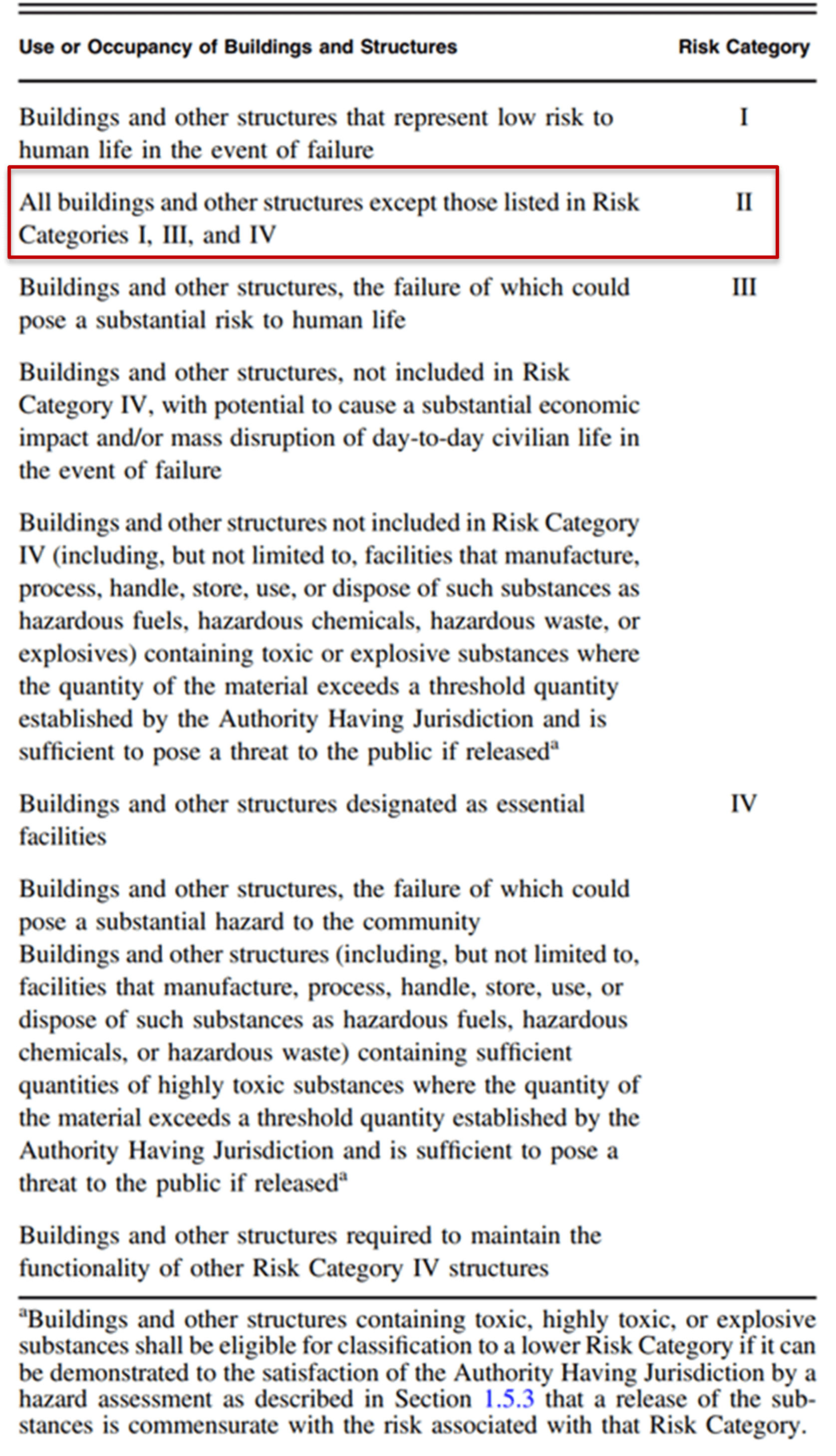### Step 2: Determine the Basic Wind Speed

The basic wind speed can be determined using the ASCE 7 Hazard Tool or by using wind speed maps provided by ASCE 7-16 Figure 26.5-1A to D and Figure 26.5-2A to D (see Figure 1), or local meteorological records.

In this example, the basic wind speed is taken as V = 89.1 mph.

### Step 3: Determine the Exposure Category

The exposure category is determined as C in accordance with section 26.7 in the ASCE 7-16.

### Step 4: Determine the Velocity Pressure Coefficients ($K_z$ and $K_h$)

The velocity pressure coefficient can be determined in two ways, as outlined in Table 2.

The first approach is by directly reading the value in the table corresponding to the height above the ground and exposure category and then interpolating from the table when the height is between the two.

In this case, the height above the ground is 36.7 ft. Hence, it is between 30 ft and 40 ft, corresponding to 0.98 and 1.04, respectively. By directly interpolating between these values, the Kz can be found as almost 1.02.

Alternatively, the equation below the table can be used to calculate the Kz. In this case, the gradient height and the exponent related to the ground surface roughness in Table 3 should first be obtained in accordance with the exposure category.

In this example the $α$ = 9.5 and $Z_g$ = 900 ft. Hence:

$K_z = 2.01(36.7/900)^{2/9.5} = 1.024 ≈ 1.02$

Table 2: Velocity pressure exposure coefficients as provided by Table 26.10-1 on ASCE 7-16 (Reference).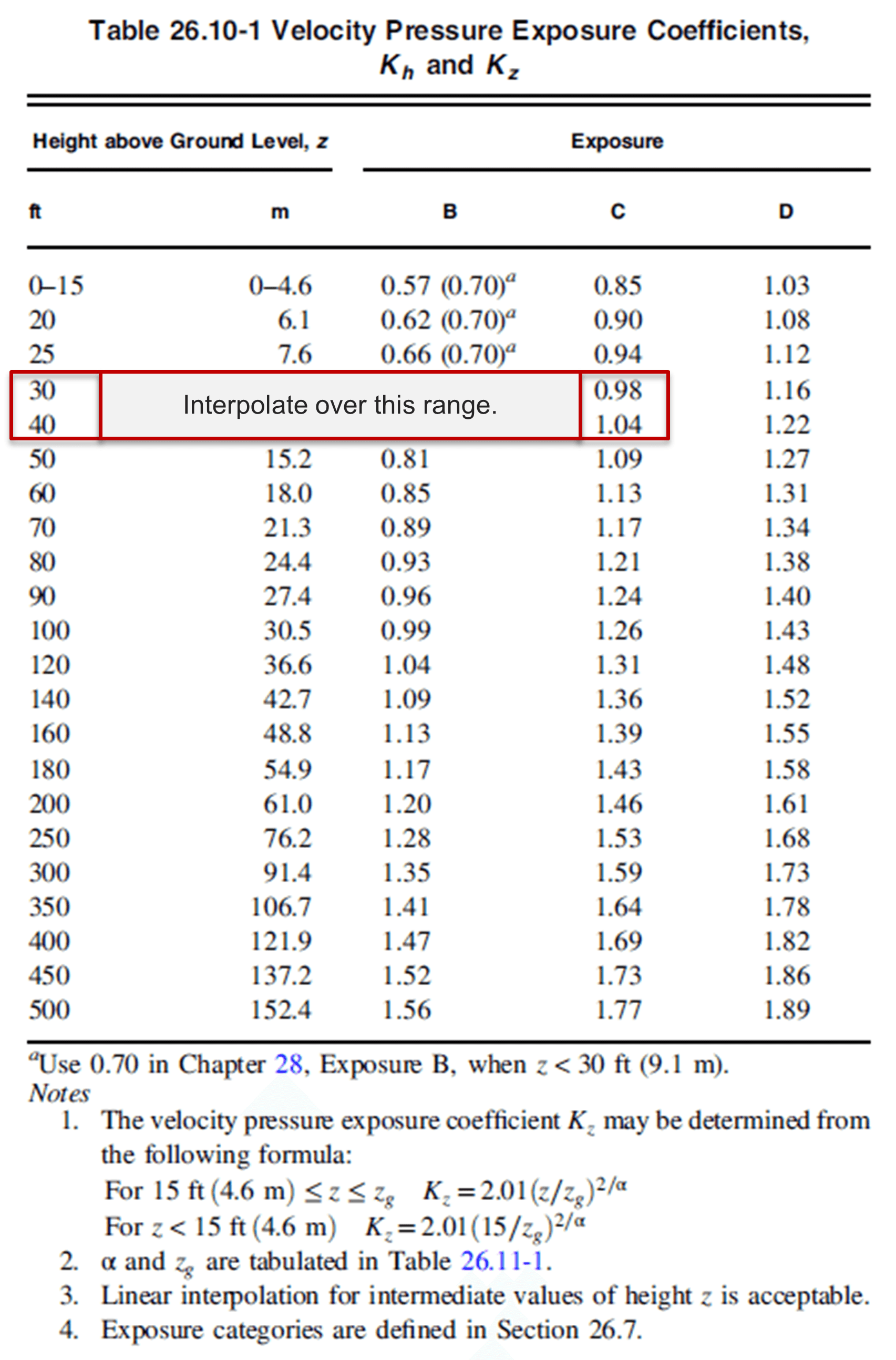Table 3: Terrain exposure constants as provided by Table 26.11-1 on ASCE 7-16 (Reference).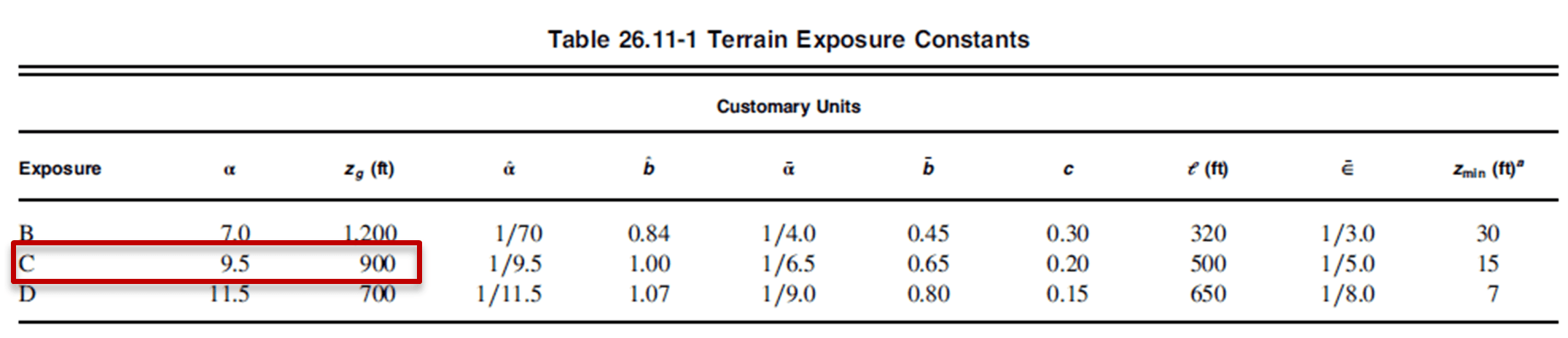### Step 5: Determine the Topographic Factor ($K_{zt}$)

The topographic factor and its multipliers can be determined using the equation and table provided in Figure 2.

In this example, H = 80 ft, Lh = 100 ft, x = 50 ft, and the modified Lh (as per the requirements below the table) = 160 ft.

Hence, the multipliers of the topographic factor can be interpolated from the table using the above values for the 2D Ridge case as follows:

At $H/L_h = 0.5, K1 = 0.72$ At $x/L_h = 0.31, K2 = 0.80$ At $Z/L_h = 0.23, K3 = 0.51$ $K_{zt} = (1+K_1K_2K_3)^2 = (1+0.72×0.8×0.51)^2 = 1.67$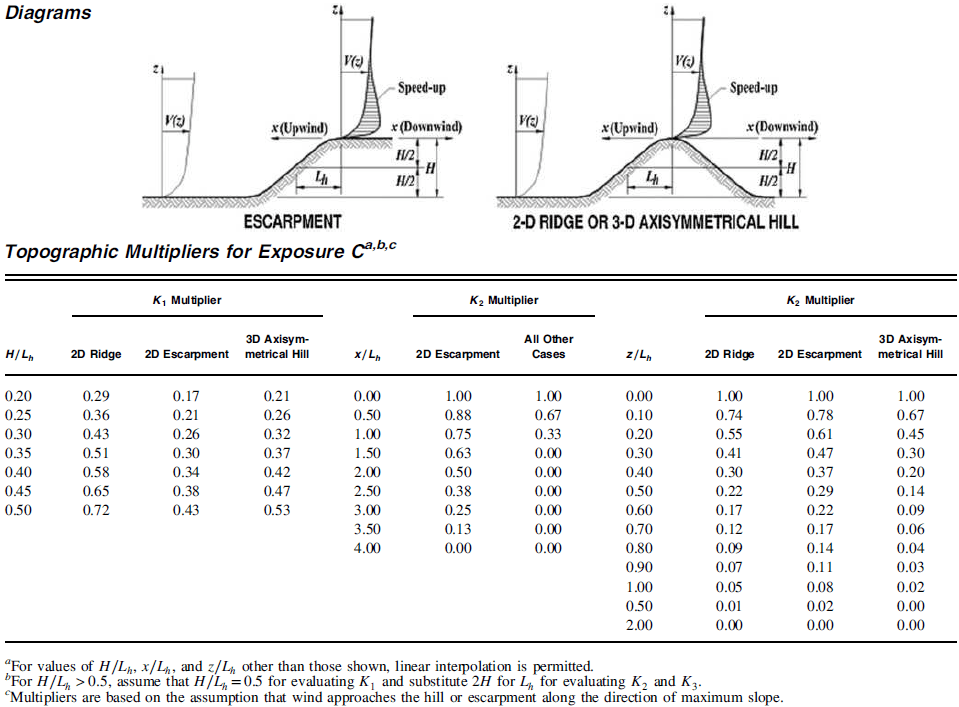Figure 2: Topographic factor and its multipliers as provided by Figure 26.8-1 on ASCE 7-16 (Reference).

### Step 6: Determine the Wind Directionality Factor ($K_d$)

The wind directionality factor can be determined using Table 4. Accordingly, Since the structural type is a building with MWFRS the $K_d$ for this example is 0.85.

Table 4. Wind directionality factor as provided by Table 26.6-1 on ASCE 7-16 (Reference).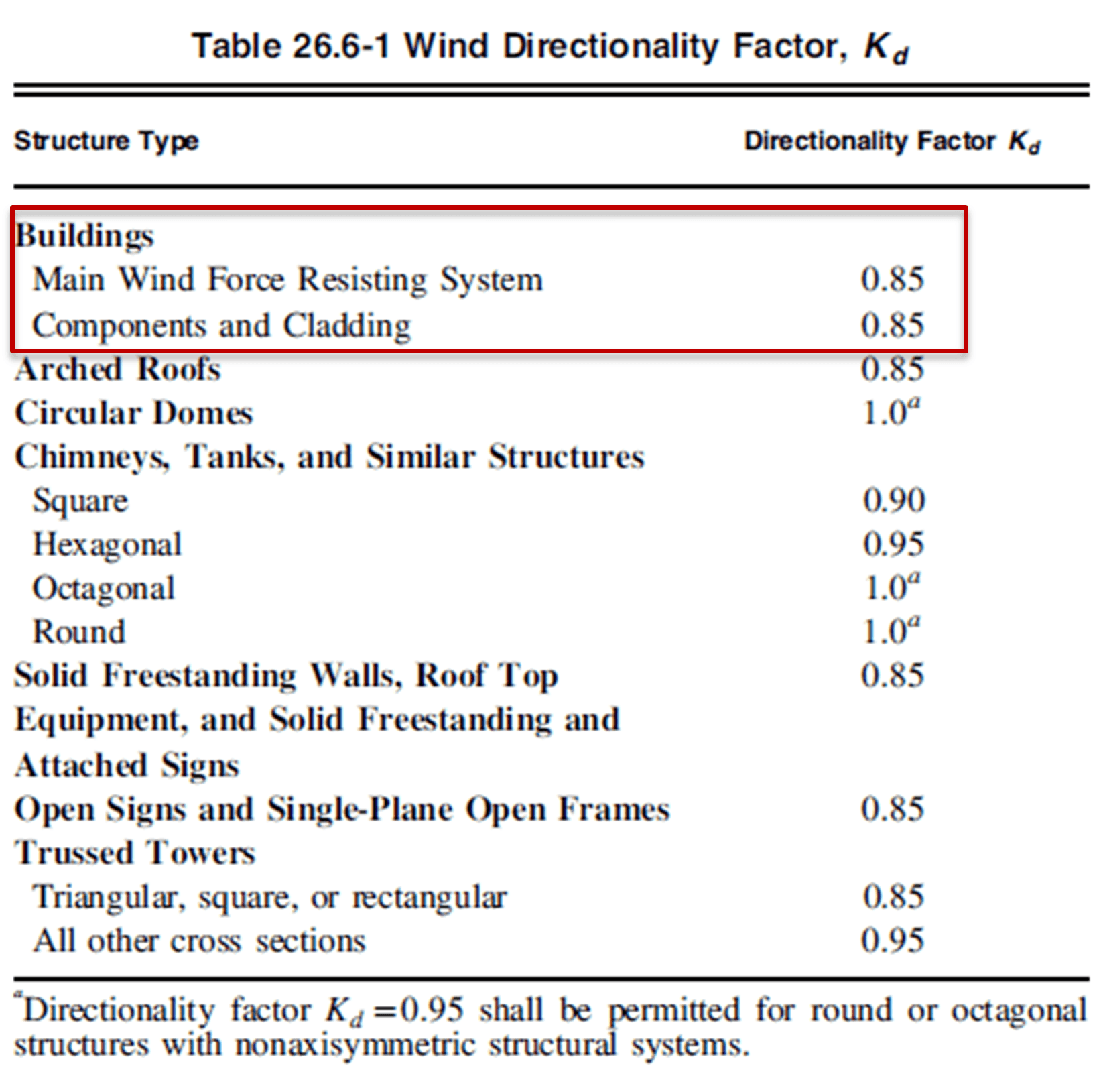### Step 7: Determine the Ground Elevation Factor ($K_e$)

The ground elevation factor can be determined from Table 5. In this example, $K_e$ is taken as 1 since the building is located at sea level.

Table 5. Ground elevation factor as provided by Table 26.9-1 on ASCE 7-16 (Reference).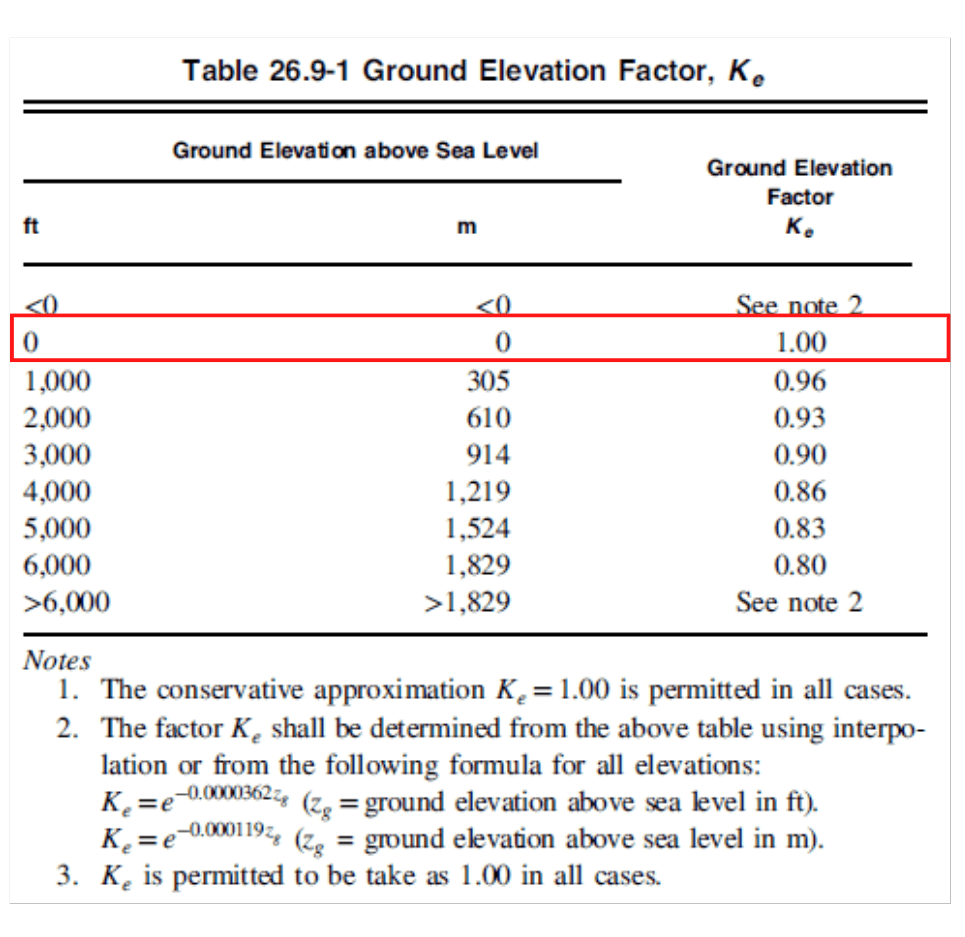### Step 8: Determine the Velocity Pressure ($q_z$)

The velocity pressure is given by the formula: $q_z=0.00256×K_z×K_zt×K_d×K_e×V^2$ $q_z = 0.00256×1.02×1.67×0.85×1×89.1 = 29.43 psf$

### Step 9: Obtain the External Pressure Coefficients for Case A and Case B ($C_p$)

The ASCE 7-16 provides various tables that can be used to obtain the pressure coefficients at different surfaces based on the building's height and roof angle.

An example of such tables can be seen in Figure 3.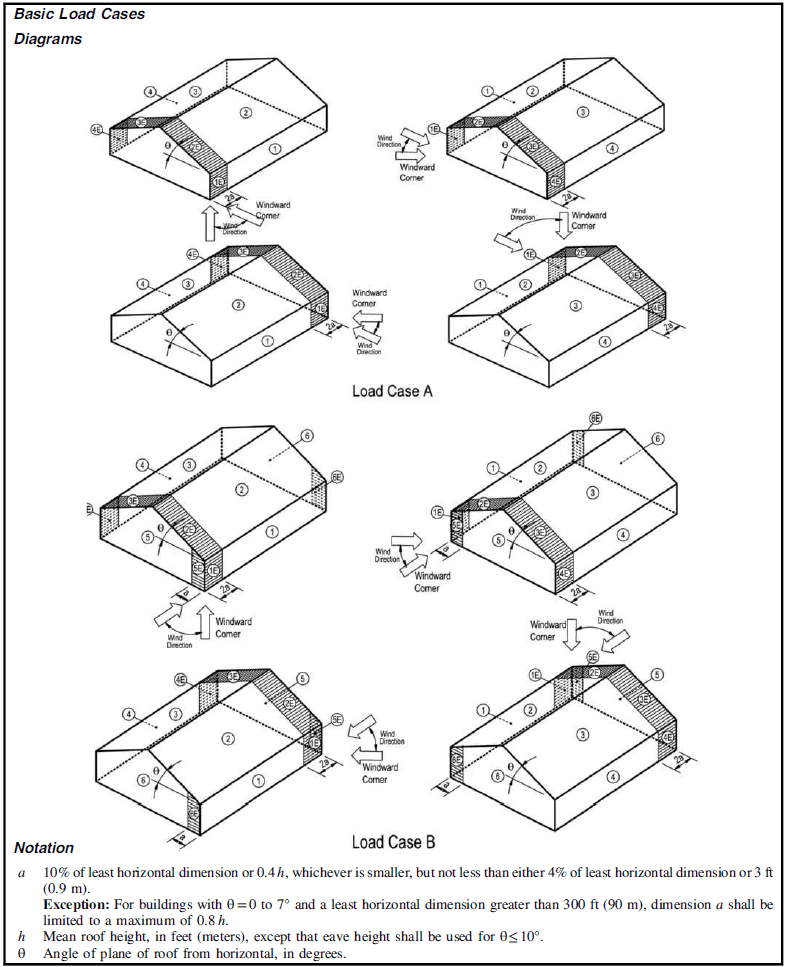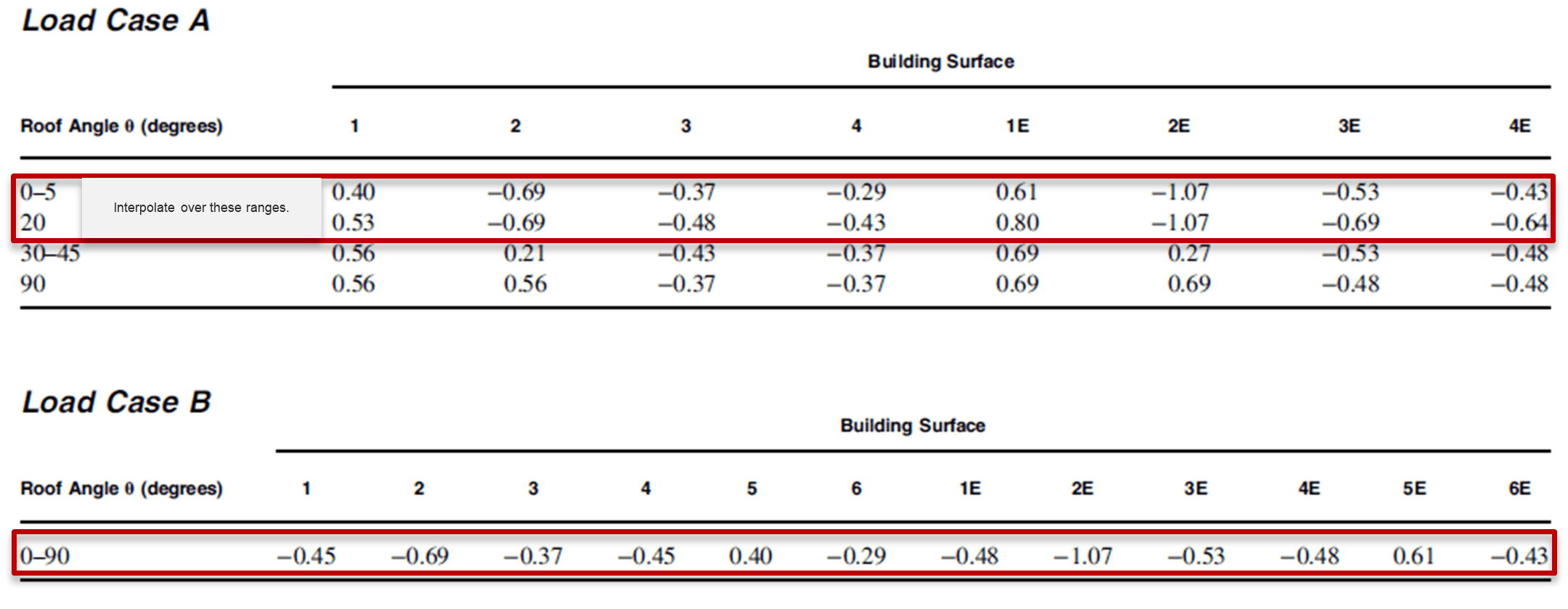Figure 3. Main wind force resisting system, part 1 [h ≤ 60 ft (h ≤ 18.3 m)]: external pressure coefficients for enclosed and partially enclosed buildings—low-rise walls and roofs.

The pressure coefficients should be collected for each of the surfaces (1 to 4 and 1E to 4E for case A and 1 to 6 and 1E to 6E for case B).

Accordingly, the pressure coefficients for load case A and B are as follows:

10.52-0.45
2-0.69-0.69
3-0.47-0.37
4-0.42-0.45
5-0.4
6--0.29
1E0.78-0.48
2E-1.07-1.07
3E-0.67-0.53
4E-0.62-0.48
5E-0.61
6E--0.43

### Step 10: Obtain the Internal Pressure Coefficient ($C_{pi}$)

The internal pressure coefficient is then obtained using Table 6. For this example the internal pressure coefficient is Cpi = ±0.18.

Table 6. Main wind force resisting system and components and cladding (all heights): internal pressure coefficient for enclosed, partially enclosed, partially open, and open buildings (walls and roof) (Reference).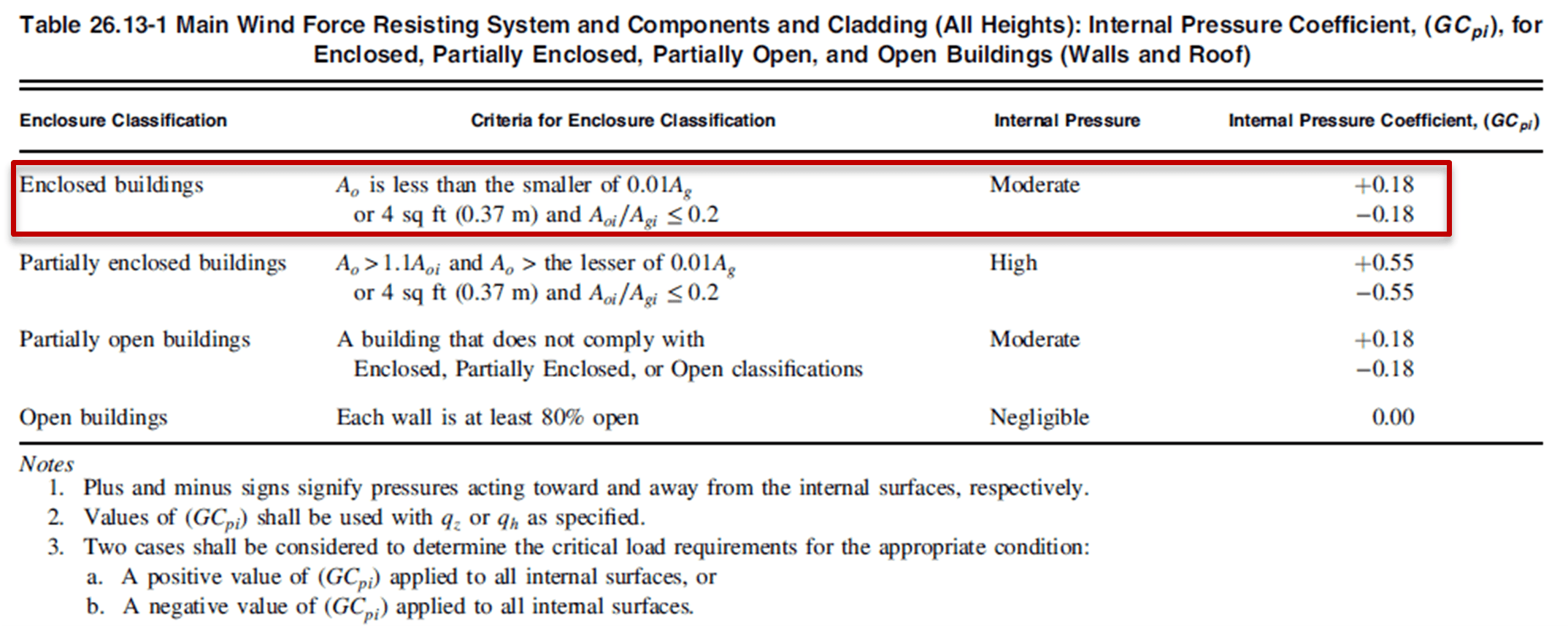### Step 11: Calculate the Design Wind Pressure for Low-Rise Buildings ($p$)

The design wind pressure for low-rise buildings can be determined using the following equation:

$p=q_z×(Cp-C_{pi})$

The detailed calculation of the design wind pressure in psf for each of the surfaces in the load case A is as follows:

Building SurfacePositive Wind PressureNegative Wind Pressure
1p = 29.43×(0.52-0.18) = 9.9p = 29.43×(0.52+0.18) = 20.5
2p = 29.43×(-0.69-0.18) = -25.6p = 29.43×(-0.69+0.18) = -15
3p = 29.43×(-0.47-0.18) = -19.1p = 29.43×(-0.47+0.18) = -8.5
4p = 29.43×(-0.42-0.18) = -17.6p = 29.43×(-0.42+0.18) = -7
1Ep = 29.43×(0.78-0.18) = 17.6p = 29.43×(0.78+0.18) = 28.2
2Ep = 29.43×(-1.07-0.18) = -36.8p = 29.43×(-1.07+0.18) = -26.2
3Ep = 29.43×(-0.67-0.18) = -25p = 29.43×(-0.67+0.18) = -14.4
4Ep = 29.43×(-0.62-0.18) = -23.5p = 29.43×(-0.62+0.18) = -12.9

For load case B, the design wind pressures in psf are as follows:

Building SurfacePositive Wind PressureNegative Wind Pressure
1p = 29.43×(-0.45-0.18) = -18.5p = 29.43×(-0.45 +0.18) = -7.95
2p = 29.43×(-0.69-0.18) = -25.6p = 29.43×(-0.69+0.18) = -15
3p = 29.43×(-0.37-0.18) = -16.2p = 29.43×(-0.37+0.18) = -5.6
4p = 29.43×(-0.45-0.18) = -18.5p = 29.43×(-0.45+0.18) = -7.9
5p = 29.43×(-0.4-0.18) = 6.48p = 29.43×(0.4+0.18) = 17
6p = 29.43×(-0.29-0.18) = -13.8p = 29.43×(-0.29+0.18) = -3.24
1Ep = 29.43×(-0.48-0.18) = -19.4p = 29.43×(-0.48+0.18) = -8.83
2Ep = 29.43×(-1.07-0.18) = -36.7p = 29.43×(-1.07+0.18) = -26.2
3Ep = 29.43×(-0.53-0.18) = -20.9p = 29.43×(-0.53+0.18) = -10.3
4Ep = 29.43×(-0.48-0.18) = -19.4p = 29.43×(-0.48+0.18) = -8.83
5Ep = 29.43×(0.61-0.18) = -12.6p = 29.43×(0.61+0.18) = 23.2
6Ep = 29.43×(-0.43-0.18) = -17.9p = 29.43×(-0.43+0.18) = -7.36

On the other hand, the process for using ClearClacs's tool is very simple given that it only requires inputting the key parameters of the terrain properties and building location and its dimensions, and the results of the computations are automatically addressed.

Finally, the calculations are compared to Struware to ensure the reliability of the results. In general, Table 7 indicates a great match between the results of all approaches.

Table 7. Comparison of the MWFRS wind loads using various approaches.

#### Case A

Positive Wind PressureNegative Wind Pressure
SurfaceManual CalculationClearCalcsStruwareManual CalculationClearCalcsStruware
Surface 19.99.99.920.420.520.5
Surface 2-25.6-25.6-25.7-15-15-15
Surface 3-19.1-19.1-19.1-8.5-8.48-8.5
Surface 4-17.6-17.5-17.6-7.0-6.92-6.9
Surface 1E17.617.617.728.228.228.3
Surface 2E-36.8-36.7-36.9-26.2-26.2-26.2
Surface 3E-25-25.1-25.2-14.4-14.5-14.5
Surface 4E-23.5-23.5-23.5-12.9-12.9-12.9

#### Case B

Positive Wind PressureNegative Wind Pressure
SurfaceManual CalculationClearCalcsStruwareManual CalculationClearCalcsStruware
Surface 1-18.5-18.5-18.6-7.95-7.93-8
Surface 2-25.6-25.6-25.7-15-15-15
Surface 3-16.2-16.2-16.2-5.6-5.58-5.6
Surface 4-18.5-18.5-18.6-7.9-7.93-8
Surface 56.486.476.5171717.1
Surface 6-13.8-13.8-13.9-3.24-3.23-3.2
Surface 1E-19.4-19.4-19.5-8.83-8.82-8.8
Surface 2E-36.7-36.7-36.9-26.2-26.2-26.2
Surface 3E-20.9-20.9-20.9-10.3-10.3-10.3
Surface 4E-19.4-19.4-19.5-8.83-8.82-8.8
Surface 5E12.612.612.723.223.223.3
Surface 6E-17.9-17.9-18-7.36-7.35-7.4

The procedure for manually calculating the wind loads in components and cladding is detailed as follows:

Repeat Step 1-8 as outlined above.

### Step 9: Obtain the External Pressure Coefficients for Case A and Case B ($C_p$)

The ASCE 7-16 provides various tables that can be used to obtain the pressure coefficients for different cases, including walls, gable roofs, stepped roofs, etc.

An example of such tables can be seen in Figure 4.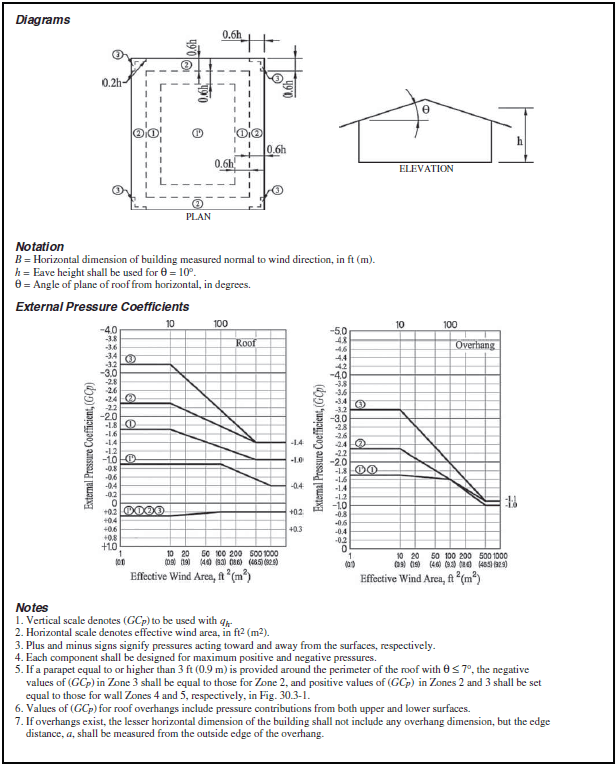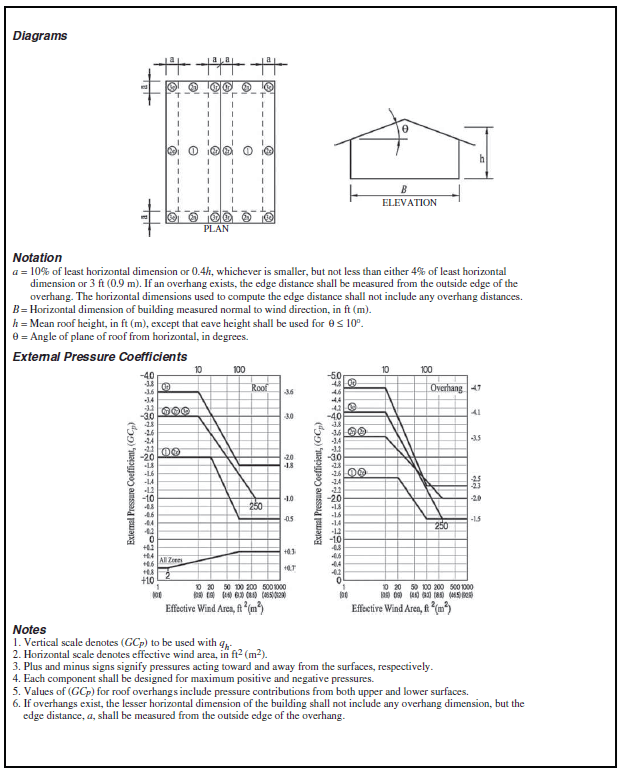Figure 4. Components and cladding [h ≤ 60 ft (h ≤ 18.3 m)]: external pressure coefficients for enclosed and partially enclosed buildings—gable roofs, θ ≤ 7°.

The pressure coefficients should be collected for each of the surfaces (1 to 4 and 1E to 4E for case A and 1 to 6 and 1E to 6E for case B).

### Step 10: Obtain the Internal Pressure Coefficient ($C_{pi}$)

The internal pressure coefficient is then obtained using Table 6. For this example the internal pressure coefficient is Cpi = ±0.18.

Table 6. Main wind force resisting system and components and cladding (all heights): internal pressure coefficient for enclosed, partially enclosed, partially open, and open buildings (walls and roof) (Reference).### Step 11: Calculate the Design Wind Pressure for Low-Rise Buildings ($p$)

The design wind pressure for low-rise buildings can be determined using the following equation: $p=q_z×(Cp-C_{pi})$

A detailed calculation of the design wind pressure in psf is provided as follows:

Building SurfacePositive Wind PressureNegative Wind Pressure
Zone 1p = 29.43×(0.7+0.18) = 25.9p = 29.43×(-2-0.18) = -64.1
Zone 2Ep = 29.43×(0.7+0.18) = 25.9p = 29.43×(-2-0.18) = -64.1
Zone 2Np = 29.43×(0.7+0.18) = 25.9p = 29.43×(-3-0.18) = -93.6
Zone 2Rp = 29.43×(0.7+0.18) = 25.9p = 29.43×(-3-0.18) = -93.6
Zone 3Ep = 29.43×(0.7+0.18) = 25.9p = 29.43×(-3-0.18) = -93.6
Zone 3Rp = 29.43×(0.7+0.18) = 25.9p = 29.43×(-3.6-0.18) = -111.2
Zone 4p = 29.43×(1+0.18) = 34.7p = 29.43×(-1.1-0.18) = -37.7
Zone 5p = 29.43×(1+0.18) = 34.7p = 29.43×(-1.4-0.18) = -46.5

In the case of calculating the components and cladding wind loads, the procedure described before was applied and compared to the automated tool in ClearCalcs.

In general, the results in Table 8 indicate a great matching among all building surfaces.

Table 8. Comparison of the components and cladding wind loads using various approaches.

Positive Wind PressureNegative Wind Pressure
SurfaceManual CalculationClearCalcsStruwareManual CalculationClearCalcsStruware
Zone 125.925.926-64.1-64.1-64.3
Zone 2E25.925.926-64.1-64.1-64.3
Zone 2N25.925.926-93.6-93.5-93.8
Zone 2R25.925.926-93.6-93.5-93.8
Zone 3E25.925.926-93.6-93.5-93.8
Zone 3R25.925.926-111.2-111-111.5
Zone 434.734.734.8-37.7-37.6-37.8
Zone 534.734.734.8-46.5-46.4-46.6

## ClearCalcs - A Better Alternative for Wind Load Analysis

As an alternative to performing the wind load calculation and analysis manually by hand, structural analysis software like the ClearCalcs wind load analysis calculator can be a time-saving tool for structural engineers and architects.

Here are some of the ClearCalcs features you can use when analyzing wind loads:

• Compliant with applicable building codes: ClearCalcs provides comprehensive wind analysis based on the wind load provisions of ASCE 7-16. Future code updates are usually integrated into the platform as soon as they are adopted by local jurisdictions and standards like the International Building Code (IBC).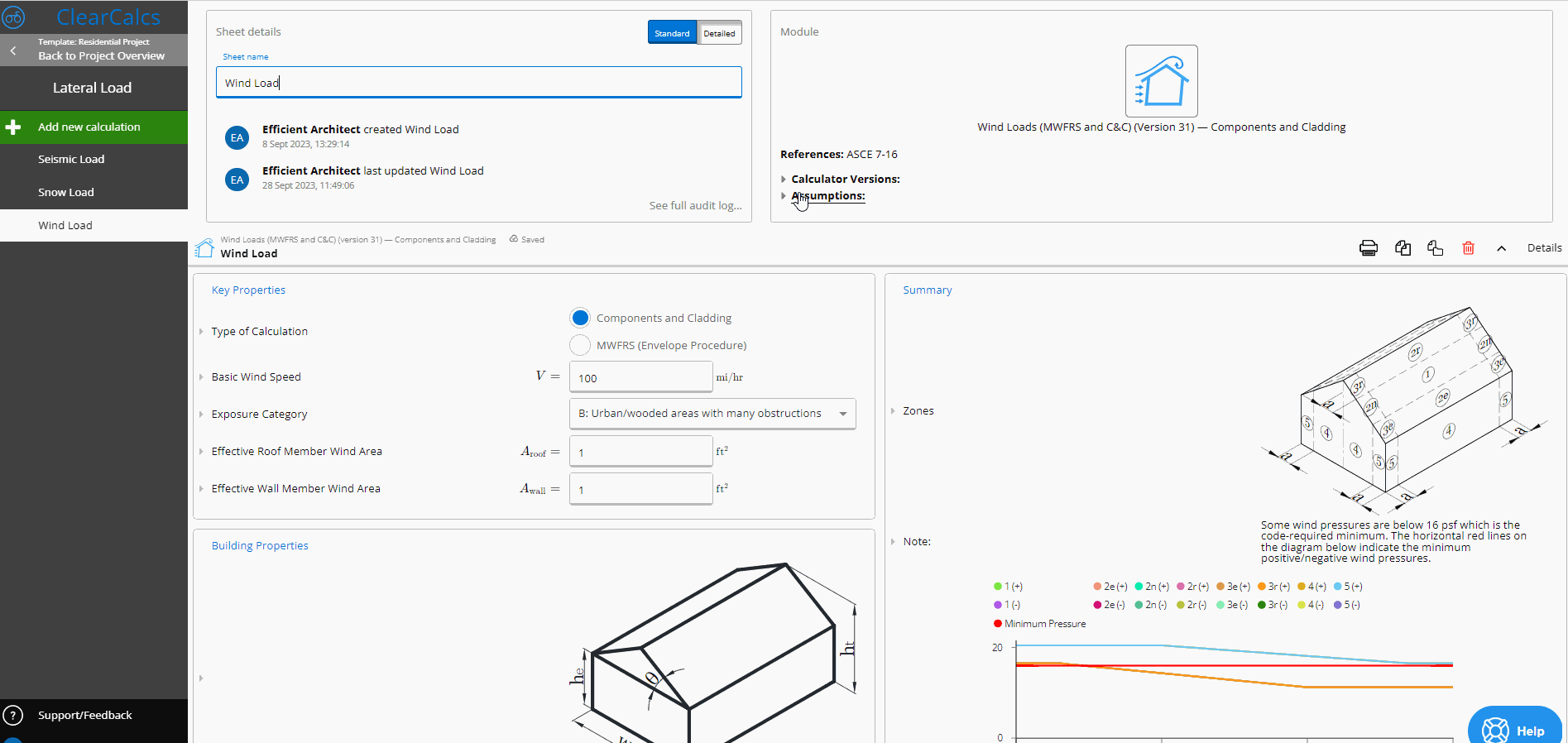• Simplified manual lookup and data input: ClearCalcs significantly simplifies laborious tasks by saving you the time from the tedious manual lookup, data input, and reference to tables by automatically inputting the formula and calculations based on your building’s geometry and key criteria like Exposure Category.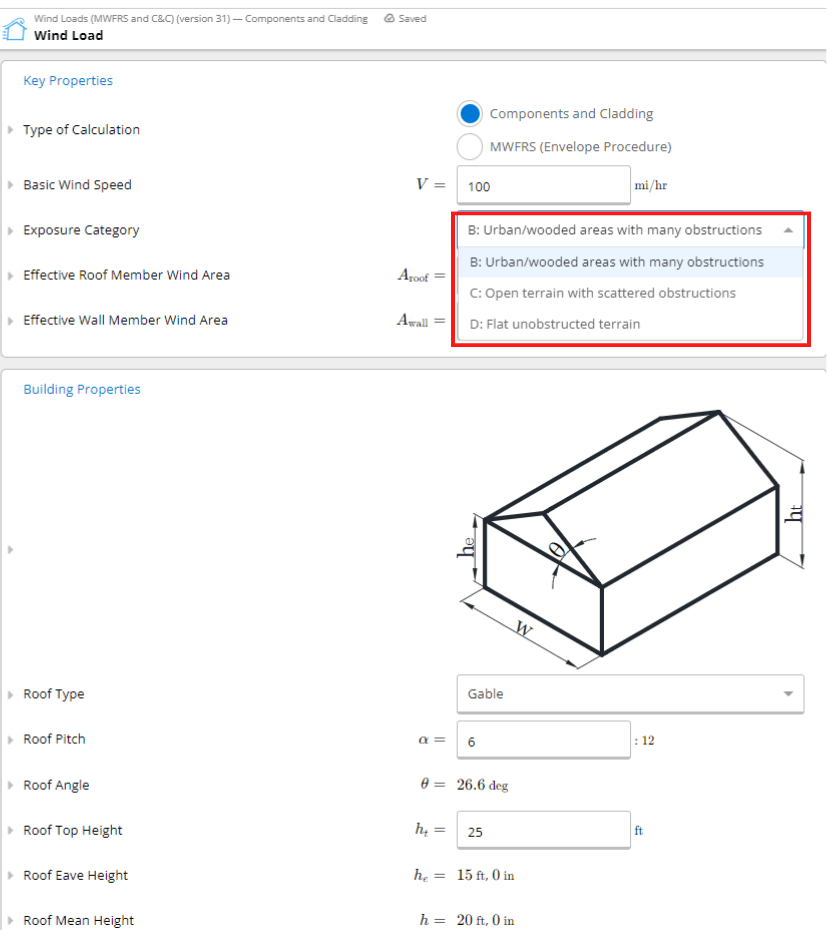• Transparent calculations: In structural calculations, doubts can arise. With ClearCalcs, users can effortlessly access relevant standards, clauses, and the logic behind specific formulas or methodologies. This ensures that the user is always informed and confident in the results produced.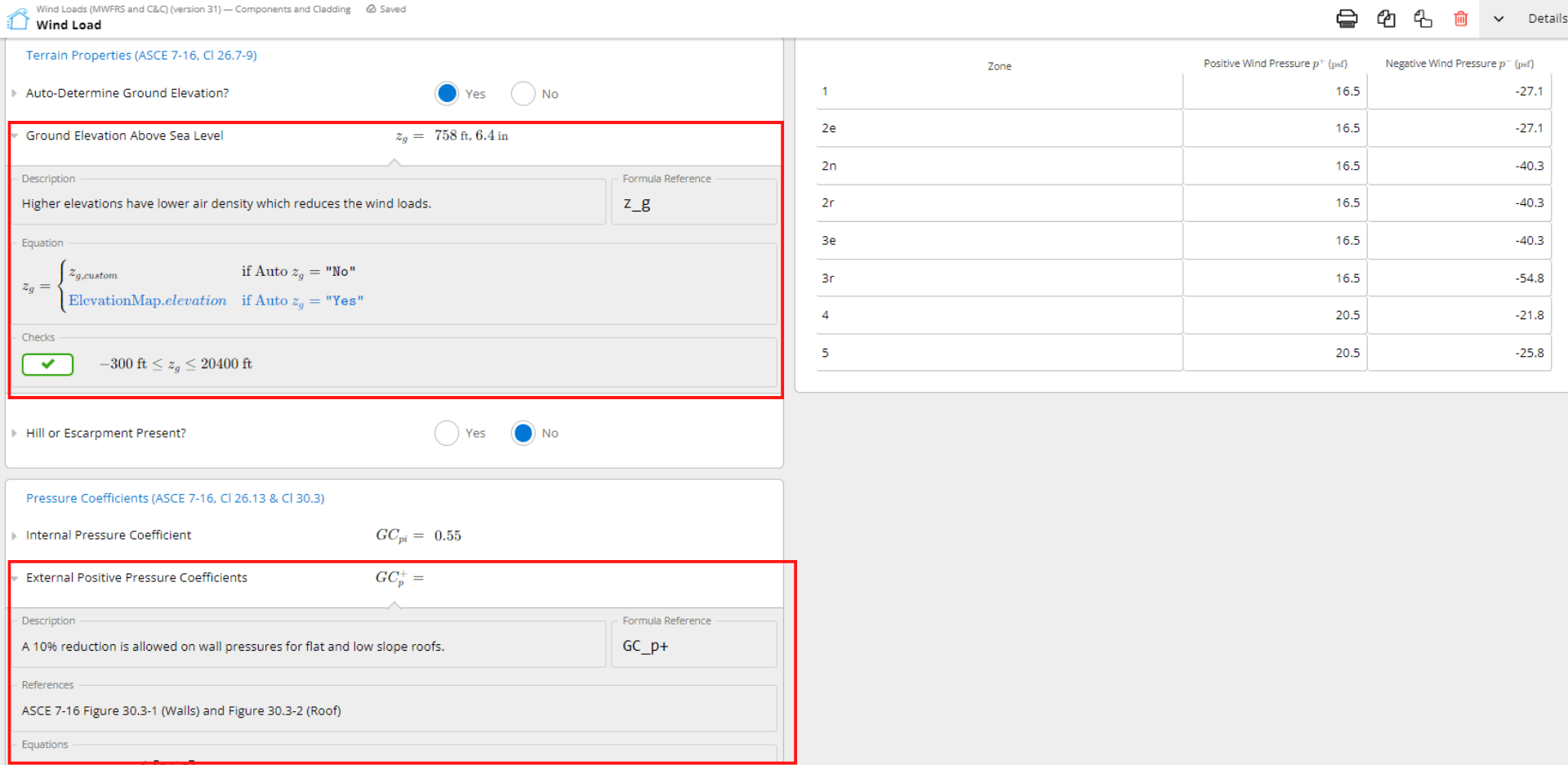• In-depth analysis: ClearCalcs provides a comprehensive and easy-to-use tool that supports simple or complex site criteria. The calculator evaluates the site’s geographical and topographical peculiarities like the presence of a hill or escarpment to ensure a comprehensive analysis and automatically calculates the ground elevation above sea level based on the building’s key properties.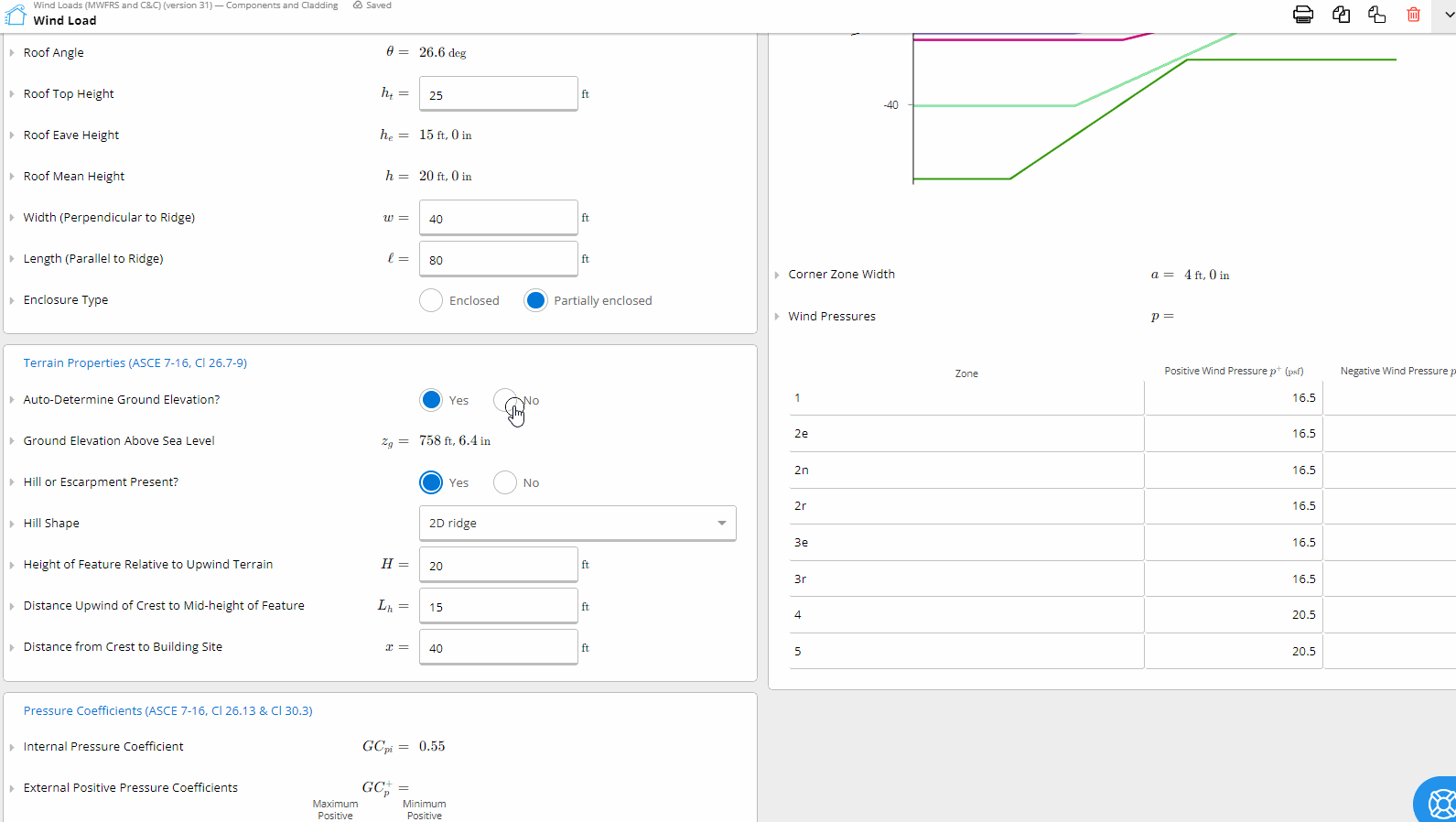Hand calculations, while tried and tested, are labor-intensive, susceptible to human errors, and consume significant time. With ClearCalcs, structural design professionals can achieve in minutes what might traditionally take hours.

## Conclusion:

When it comes to structural engineering, accurate wind load calculations are crucial for ensuring the safety, integrity, and durability of buildings. These assessments are necessary to determine if a structure can withstand external pressures, and they play a crucial role in achieving cost-effective and resilient design strategies.

Recent numerical examples have shown that both the ClearCalcs methodology and the traditional manual approach produce similar results. However, the key difference lies in the efficiency and user-friendliness of the processes. While manual calculations may be more accurate, ClearCalcs can achieve comparable precision in a shorter amount of time, reducing the need for manual labor and the potential for human errors. Additionally, ClearCalcs' intuitive design makes it easy for individuals with limited technological expertise to navigate its features.

By incorporating tools like ClearCalcs, structural engineers and architects have the opportunity to combine the reliability of traditional methods with the speed and efficiency of modern technology. This tool represents a fusion of precision and practicality, demonstrating the convenience and effectiveness of contemporary innovations in the structural engineering industry.

Structural engineers and architects now have access to advanced tools that improve their accuracy and efficiency, making this an exciting and transformative time for their field.

#### Ready to start calculating in ClearCalcs?

You can use the account for 14 days for free, with no commitment required. Need ClearCalcs for a one-off project? Subscribe to our month-to-month plan and cancel anytime, no questions asked.